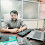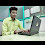# Break,Continue and Pass Statement in Python

16

Break, Continue and Pass Statement in Python:-

Break:-   It is used to manually terminate the loop based on condition, the break statement always will be defined by the Conditional Statement in Loop.
for i in range(1,10):
if i==3:
break;
print(i)
output:-  1
2
Continue:-   It is used to skip the particular data from the loop and continue the process of the loop.
for i in range(1,10):
if i==3:
continue
print(i)
output:-  1
2
4
5
6
7
8
9
the important question of break and continue:-
for i in range(1,10):
if i==3 and i==5:
continue
print(i)
print("hello")
..................................
for i in range(1,10):
if i==3 and i==5:
break
print(i)
print("hello")

for i in range(1,10):
if i==3 or i==5:
continue
print(i)
print("hello")
........................................................................
pass:-

it is used to define an empty block in python because python does not use braces means if we want to only define method structure, a loop structure, if-else structure empty then we can use pass statement
for(i=1;i<=10;i++)   #C empty loop
{
}
for i in range(1,10):
pass
Assignment of Loop:-
WAP to display the salary of employees where empid will be started from 1001 to 1020.
empid 1003,1005 and 1007 salary should not be displayed,empid 1013 and 1015,2 days salary should be deduced, if the entered salary is above 20000 then the calculation will be performed till 1017.
A salary is common for all employees.

Tags

1."""WAP to display the salary of employees where empid will be started from 1001 to 1020.
empid 1003,1005 and 1007 salary should not be displayed,empid 1013 and 1015,2 days salary should be deduced, if the entered salary is above 20000 then the calculation will be performed till 1017.
A salary is common for all employees.
"""

salary=30000
perdaysalary=salary//30
p=perdaysalary
for i in range(1001,1021):
if i==1003 or i==1005 or i==1007:
continue

print("empid",i,end=''+"salary="+str(salary)+'\n')

if i==1013 :
print("empid",i,end=''+"salary="+str(salary-p)+'\n')
elif i==1015:
print("empid",i,end=''+"salary="+str(salary-p)+'\n')

s=int(input("enter salary"))
for j in range(1001,1018):
if s>20000:
print("empid",j,end=''+"salary="+str(s)+'\n')

else:
print("salary less than 20000")

print()

1.This will be much better as compare to that program
sal = int(input("enter salary of employee"))
for empid in range(1001,1021):
if sal>=20000 and empid>1017:
break
if empid==1003 or empid==1005 or empid==1007:
continue
elif empid==1013 or empid==1015:
dsal = (sal/30)*2
print("empid ",empid, "salary is ",sal-dsal)

else:
print("empid ",empid, "salary is ",sal)

2.Program to display the salary of employees where empid will be started from 1001 to 1020.empid 1003,1005 and 1007 salary should not be displayed,empid 1013 and 1015,2 days salary should be deduced, if the entered salary is above 20000 then the calculation will be performed till 1017---->>

sal=int(input("enter salary of employee"))
pds=sal/30 #pds=per day salary
rsal=sal-pds*2
for empid in range(1001,1021):
if sal>20000 and empid>1017:
break
if empid==1003 or empid==1005 or empid==1007:
continue
elif empid==1013 or empid==1015:
rsal=sal-pds*2
print("empid",empid,"salary is",rsal)
else:
print("empid",empid,"sal is",sal)

3.Sir in my program output is--
enter salary of employee13654
empid 1001 salary is 12743.733333333334
empid 1002 salary is 12743.733333333334
empid 1004 salary is 12743.733333333334
empid 1006 salary is 12743.733333333334
empid 1008 salary is 12743.733333333334
empid 1009 salary is 12743.733333333334
empid 1010 salary is 12743.733333333334
empid 1011 salary is 12743.733333333334
empid 1012 salary is 12743.733333333334
empid 1014 salary is 12743.733333333334
empid 1016 salary is 12743.733333333334
empid 1017 salary is 12743.733333333334
empid 1018 salary is 12743.733333333334
empid 1019 salary is 12743.733333333334
empid 1020 salary is 12743.733333333334

which is not according to given condition,in output empid-1013 and 1015's salary should be reduced but it is not executed in output.Both these empid is not executing in output.What is the correction?

4.WAP to display the salary of employees where empid will be started from 1001 to 1020.
empid 1003,1005 and 1007 salary should not be displayed,empid 1013 and 1015,2 days salary should be deduced, if the entered salary is above 20000 then the calculation will be performed till 1017.
A salary is common for all employees.
start = 1001
end = 1020
salary =int(input("Enter salary = "))

for empid in range(start, end+1):
if empid == 1003 or empid==1005 or empid == 1007:
continue
elif empid == 1013 or empid == 1015:
x =salary/30
l = 2*x
print(str(empid)+ ' = ' + str(salary-l) +str(" leave has been deducted"))
elif salary >= 20000 and empid == 1017:

print(empid,"=",salary)
break

else:
print(empid,"=",salary)

5.#MOHIT CHOUHAN

for empid in range(1001,1021):

if salary>20000 and empid>1017:
break
if empid==1003 or empid==1005 or empid==1007:
continue
if empid==1013 or empid==1015:
day=(salary/30)*2
Deduct=salary-day
print(empid,Deduct)
else:
print(empid,salary)

6.#Vishal Baghel#

salary = int(input("Enter Employees Salary = "))
for i in range(1001,1021):
if i>1017 and salary>20000:
break
if i==1003 or i==1005 or i==1007:
continue
elif i==1013 or i==1015:
p_day = salary//30
print("Employee_ID = ",i,"Salary After 2 Days Salary Deduced = ",salary-p_day*2)
else:
print("Employee_ID = ",i,"Salary is = ",salary )

7.#Shivam Shukla
#1.1) Assignment Solution
sal=int(input("Enter a Salary : "));
cutsal=sal-((sal/30)*2);
s = 1018 if(sal>20000) else 1021;
for i in range(1001,s):
if(i==1003 or i==1005 or i==1007):
continue;
if(i==1013 or i==1015):
print("EmpId :",i,cutsal);
continue;
print("EmpId :",i,sal);

8.#Shivam Shukla
#1.2) Assignment Solution
sal=int(input("Enter a Salary : "));
cutsal=sal-((sal/30)*2);
for i in range(1001,1021):
if(sal>20000 and i==1018):
break;
if(i==1003 or i==1005 or i==1007):
continue;
if(i==1013 or i==1015):
print("EmpId :",i,cutsal);
continue;
print("EmpId :",i,sal);

9.sal=int(input("enter a number"))
ds=sal//30
if sal>20000:
for i in range(1001,1018):
if i==1013 or i==1015:
print(i," salary is",sal-(ds*2))
if i==1003 or i==1005 or i==1007 or i==1013 or i==1015:
continue;
print(i,"salary is",sal)
if sal<20000:
for i in range(1001,1021):
if i==1003 or i==1005 or i==1007:
continue;
print(i,"salary is",sal)

10.WAP to display the salary of employees where empid will be started from 1001 to 1020.
empid 1003,1005 and 1007 salary should not be displayed,empid 1013 and 1015,2 days salary should be deduced, if the entered salary is above 20000 then the calculation will be performed till 1017.
A salary is common for all employees.

salary = int(input("enter salary of employee : "))
for emyid in range(1001,1021):
if salary>=20000 and emyid>1017:
break
if emyid==1003 or emyid==1005 or emyid==1007:
continue
if emyid==1013 or emyid==1015:
deducteddsal = (salary/30)*2
print("emyid ",emyid, "salary is % .2f " % (salary-deducteddsal))
else:
print("emyid ",emyid, "salary is ",salary)

11.salary=int(input("Enter salary "))
for i in range(1001,1021):
if salary>20000 and i>1017:
break
elif i==1003 or i==1005 or i==1007:
continue
elif i==1013 or i==1015:
dsalary=salary-(2*(salary//30))
print("EmpID "+str(i)+" salary after deduction "+str(dsalary))

else:
print("EmpID "+str(i)+" salary "+str(salary))

12.'''WAP to display the salary of employees where empid will be started from 1001 to 1020.
empid 1003,1005 and 1007 salary should not be displayed,empid 1013 and 1015,2 days salary
should be deduced, if the entered salary is above 20000 then the calculation will be performed till 1017.
A salary is common for all employees.'''

salary=int(input("enter salary"))
dstwodays=(salary//30)*2
for i in range(1001,1021):
if i>1017 and salary>20000:
break
if i==1003 or i==1005 or i==1007:
continue
elif i==1013 or i==1015:
print(i,"salary",salary-dstwodays)
else:
print(i,"salary",salary)

13.#WAP to display the salary of employees where empid will be started from 1001 to 1020.
empid 1003,1005 and 1007 salary should not be displayed,empid 1013 and 1015,2 days salary should be deduced, if the entered salary is above 20000 then the calculation will be performed till 1017.
A salary is common for all employees.

salary =22000
for empid in range (1001,1021):
if salary>=20000 and empid>1017:
break
if empid ==1003 or empid ==1005 or empid ==1007:
continue
elif empid==1013 or empid==1015:
dsal=(salary/30)*2
salary1=salary-dsal

print("empid ",empid,"salary is",salary1)
else:

print("empid",empid,"salary is",salary)

14.salary=int(input("enter salary"))
deduceds=(salary//30)*2
for i in range(1001,1021):
if i==1017 and salary>20000:
break
if i==1003 or i==1005 or i==1007:
continue
elif i==1013 or i==1015:
print(i,"salary",salary-deduceds)
else:
print(i,"salary",salary)

15.#continue program
for i in range(1,11):
if i==3 and i==5:
continue
print(i)
print("hello")